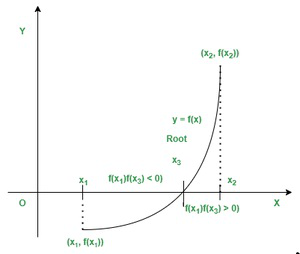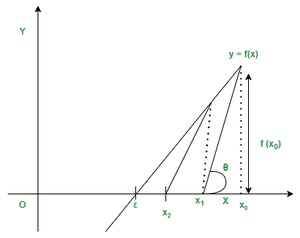Open in App
Not now

# Difference between Bisection Method and Newton Raphson Method

• Last Updated : 28 Jan, 2022

Numerical methods are the set of tasks by applying arithmetic operations to numerical equations. We can formulate mathematical problems to find the approximate result. This formulation is called the numerical implementation of the problem. In this, there is no need for algorithms. Programming logic is then developed for numerical implementation. The programming is usually done with some high-level languages like Fortran, Basic, etc.

### Bisection Method

This method is based on the repeated application of the intermediate value property. Let f(x) is continuous function in the closed interval [x1, x2], if f(x1), f(x2) are of opposite signs, then there is at least one root α in the interval (x1, x2), such that f(α) = 0.Bisection Method

Formula is: x2 = (x0 + x1) / 2

### Newton Raphson Method

The Newton Raphson Method is the process for the determination of a real root of an equation f(x)=0 given just one point close to the desired root.Newton Raphson method

Formula is: x1 = x0 – f(x0)/f'(x0)

### Comparison between Bisection Method and Newton Raphson Method

Question 1: Find a root of an equation f(x) = x3 – x – 1

Solution:

Given equation f(x) = x3 – x – 1

let x  = 0, 1, 2

• In 1st iteration:

f(1) = -1 < 0 and f(2) = 5 > 0

The root lies between these two points 1 and 2

x0 = 1 + 2/2 = 1.5

f(x0) = f(1.5) = 0.875 > 0

• In 2nd iteration:

f(1) = -1 < 0 and f(1.5) = 0.875 > 0

The root lies between these two points 1 and 1.5

x1 = 1 + 1.5/2 = 1.25

f(x1) = f(1.25) = -0.29688 < 0

• In 3rd iteration:

f(1.25) = -0.29688 < 0 and f(1.5) = 0.875 > 0

The root lies between these two points 1.25 and 1.5

x2 = 1.25 + 1.5/2 = 1.375

f(x2) = f(1.375) = 0.22461 > 0

• In 4th iteration:

f(1.25) = -0.29688 < 0 and f(1.375) = 0.22461 > 0

The root lies between these two points 1.25 and 1.375

x3 = 1.25 + 1.375/2 = 1.3125

f(x3) = f(1.3125) = -0.05151 < 0

• In 5th iteration:

f(1.3125) = -0.05151 < 0 and f(1.375) = 0.22461 > 0

The root lies between these two points 1.3125 and 1.375

x4 = 1.3125 + 1.375/2 = 1.34375

f(x4) = f(1.34375) = 0.08261 > 0

• In 6th iteration:

f(1.3125) = -0.05151 < 0 and f(1.34375) = 0.08261 > 0

The root lies between these two points 1.3125 and 1.34375

x5 = 1.3125 + 1.34375/2 = 1.32812

f(x5) = f(1.32812) = 0.01458 > 0

• In 7th iteration:

f(1.3125) = -0.05151 < 0 and f(1.32812) = 0.01458 > 0

The root lies between these two points 1.3125 and 1.32812

x6 = 1.3125 + 1.32812/2 = 1.32031

f(x6) = f(1.32031) = -0.01871 < 0

• In 8th iteration:

f(1.32031) = -0.01871 < 0 and f(1.32812) = 0.01458 > 0

The root lies between these two points 1.32031 and 1.32812

x7 = 1.32031 + 1.32812/2 = 1.32422

f(x7) = f(1.32422) = -0.00213 < 0

• In 9th iteration:

f(1.32422) = -0.00213 < 0 and f(1.32812) = 0.01458 > 0

The root lies between these two points 1.32422 and 1.32812

x8 = 1.32422 + 1.32812/2 = 1.32617

f(x8) = f(1.32617) = 0.00621 > 0

• In 10th iteration:

f(1.32422) = -0.00213 < 0 and f(1.32617) = 0.00621 > 0

The root lies between these two points 1.32422 and 1.32617

x9 = 1.32422 + 1.32617/2 = 1.3252

f(x9) = f(1.3252) = 0.00204 > 0

• In 11th iteration:

f(1.32422) = -0.00213 < 0 and f(1.3252) = 0.00204 > 0

The root lies between these two points 1.32422 and 1.3252

x10 = 1.32422 + 1.3252/2 = 1.32471

f(x10) = f(1.32471) = -0.00005 < 0

The approximate root of the equation x3 – x – 1 = 0 using the Bisection method is 1.32471

Question 2: Find a root of an equation f(x) = 2x3 – 2x – 5

Solution:

Given Equation f(x) = 2x3 – 2x – 5

• In 1st iteration:

f(1) = -5 < 0 and f(2) = 7 > 0

The root lies between these two points 1 and 2

x0 = 1 + 2/2 = 1.5

f(x0) = f(1.5) = 2 × 1.53 – 2 × 1.5 – 5 = -1.25 < 0

• In 2nd iteration:

f(1.5) = -1.25 < 0 and f(2) = 7 > 0

The root lies between these two points 1.5 and 2

x1 = 1.5 + 2/2 = 1.75

f(x1) = f(1.75) = 2 × 1.753 – 2 × 1.75 – 5 = 2.21875 > 0

• In 3rd iteration:

f(1.5) = -1.25 < 0 and f(1.75) = 2.21875 > 0

The root lies between these two points1.5 and 1.75

x2 = 1.5 + 1.75/2 = 1.625

f(x2) = f(1.625) = 2 × 1.6253 – 2 × 1.625 – 5 = 0.33203 > 0

• In 4th iteration:

f(1.5) = -1.25 < 0 and f(1.625) = 0.33203 > 0

The root lies between these two points 1.5 and 1.625

x3 = 1.5 + 1.625/2 = 1.5625

f(x3) = f(1.5625) = 2 × 1.56253 – 2 × 1.5625 – 5 = -0.49561 < 0

• In 5th iteration:

f(1.5625) = -0.49561 < 0 and f(1.625) = 0.33203 > 0

The root lies between these two points 1.5625 and 1.625

x4 = 1.5625 + 1.625/2 = 1.59375

f(x4) = f(1.59375) = 2 × 1.593753 – 2 × 1.59375 – 5 = -0.09113 < 0

• In 6th iteration:

f(1.59375) = -0.09113 < 0 and f(1.625) = 0.33203 > 0

The root lies between these two points 1.59375 and 1.625

x5 = 1.59375 + 1.625/2 = 1.60938

f(x5) = f(1.60938) = 2 × 1.609383 – 2 × 1.60938 – 5 = 0.1181 > 0

• In 7th iteration:

f(1.59375) = -0.09113 < 0 and f(1.60938) = 0.1181 > 0

The root lies between these two points 1.59375 and 1.60938

x6 = 1.59375 + 1.60938/2 = 1.60156

f(x6) = f(1.60156) = 2 × 1.601563 – 2 × 1.60156 – 5 = 0.0129 > 0

• In 8th iteration:

f(1.59375) = -0.09113 < 0 and f(1.60156) = 0.0129 > 0

The root lies between these two points 1.59375 and 1.60156

x7 = 1.59375 + 1.60156/2 = 1.59766

f(x7) = f(1.59766) = 2 × 1.597663 – 2 × 1.59766 – 5 = -0.03926 < 0

• In 9th iteration:

f(1.59766) = -0.03926 < 0 and f(1.60156) = 0.0129 > 0

The root lies between these two points 1.59766 and 1.60156

x8 = 1.59766 + 1.60156/2 = 1.59961

f(x8) = f(1.59961) = 2 × 1.599613 – 2 × 1.59961 – 5 = -0.01322 < 0

• In 10th iteration:

Here f(1.59961) = -0.01322 < 0 and f(1.60156) = 0.0129 > 0

The root lies between these two points 1.59961 and 1.60156

x9 = 1.59961 + 1.60156/2 = 1.60059

f(x9) = f(1.60059) = 2 × 1.600593 – 2 × 1.60059 – 5 = -0.00017 < 0

The Approximate root of the equation 2x3 – 2x – 5 = 0 using Bisection method is 1.60059

Question 3: Find a root of an equation f(x) = x3 – x – 1

Solution:

Given equation x3 – x – 1 = 0

Using differentiate method the equation is

∴ f′(x) = 3x2 – 1

Here f(1) = -1 < 0 and f(2) = 5 > 0

∴ Root lies between 1 and 2

x0 = 1 + 2/ 2 = 1.5

• In 1st iteration:

f(x0) = f(1.5) = 0.875

f′(x0) = f′(1.5) = 5.75

x1 = x0 – f(x0) / f′(x0)

x1 = 1.5 – 0.875/ 5.75

x1 = 1.34783

• In 2nd iteration:

f(x1) = f(1.34783) = 0.10068

f′(x1) = f′(1.34783) = 4.44991

x2 = x1 – f(x1)/f′(x1)

x2 = 1.34783 – 0.10068/4.44991

x2 = 1.3252

• In 3rd iteration:

f(x2) = f(1.3252) = 0.00206

f′(x2) = f′(1.3252) = 4.26847

x3 = x2 –  f(x2)/f′(x2)

x3 = 1.3252 – 0.00206/4.26847

x3 = 1.32472

• In 4th iteration:

f(x3) = f(1.32472) = 0

f′(x3) = f′(1.32472) = 4.26463

x4 = x3 – f(x3)/f′(x3)

x4 = 1.32472 – 0/ 4.26463

x4 = 1.32472

The Approximate root of the equation x3 – x – 1 = 0 using the Newton Raphson method is 1.32472

Question 4: Find a root of an equation f(x) = 2x3 – 2x – 5

Solution:

Given equation 2x3 – 2x – 5 = 0

Using differentiate method the equation is

∴ f′(x) = 6x2 – 2

Here f(1) = -5 < 0 and f(2) = 7 > 0

∴ Root lies between 1 and 2

x0 = 1 + 2/ 2 = 1.5

• In 1st iteration:

f(x0) = f(1.5) = 2 × 1.53 – 2 × 1.5 – 5 = -1.25

f′(x0) = f′(1.5) = 6 × 1.52 – 2 = 11.5

x1 = x0 – f(x0)/f′(x0)

x1 = 1.5 – (-1.25)/11.5

x1 = 1.6087

• In 2nd iteration:

f(x1) = f(1.6087) = 2 × 1.60873 – 2 × 1.6087 – 5 = 0.1089

f′(x1) = f′(1.6087) = 6 × 1.60872 – 2 = 13.52741

x2 = x1 – f(x1)/f′(x1)

x2 = 1.6087 – 0.1089/13.52741

x2 = 1.60065

• In 3rd iteration:

f(x2) = f(1.60065) = 2 × 1.600653 – 2 × 1.60065 – 5 = 0.00062

f′(x2) = f′(1.60065) = 6 × 1.600652 – 2 = 13.37239

x3 = x2 – f(x2)/f′(x2)

x3 = 1.60065 – 0.00062/13.37239

x3 = 1.6006

The Approximate root of the equation 2x3 – 2x – 5 = 0 using the Newton Raphson method is 1.6006

My Personal Notes arrow_drop_up
Related Articles